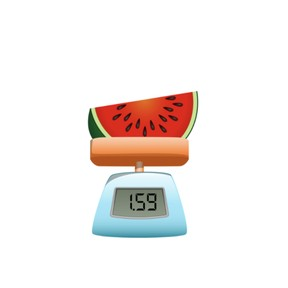Place value- rounding decimal numbers to the nearest tenth

# Place value- rounding decimal numbers to the nearest tenth8,000 schools use Gynzy92,000 teachers use Gynzy1,600,000 students use Gynzy

## General

Students learn to round decimal numbers to the nearest tenth. They use the decimal place value chart.

## Relevance

Discuss with students that learning to round to the tenths place will help speed up their calculations.

## Introduction

The interactive whiteboard shows a range of heights that one of the students has measured. They are shown as having 1 decimal place. Ask to round to see about how tall everyone is. Ask students how to round a number. When do you round up? And round down?

## Development

Explain to students that you can also round a number to the tenths place, by looking at the number after the tenths place, so the hundredths. This is the second digit after the decimal place. If the number is 4 or less, the number in the tenths place stays the same. If the number is 5 or greater, you add 1 tenth to the tenths place. You can use the decimal place value chart as support. Next you can show how to round to the tenths place by dragging the numbers to the decimal place value chart and looking at the hundredths.

Check that students understand rounding decimal numbers to the tenths place by asking the following questions:
- Which number do you look at to determine if you round up or down to the tenths place?
- What do you do if you have 5 hundredths?
- What do you do with the tenths if the number in the hundredths place is 5 or more?

## Guided practice

Students first round to the tenths place, in numbers with two and three decimal places. They have multiple options to choose from. Next students round a decimal number with 3 decimal places to the tenths place, and they need to write in the number. Ask students to explain how they determine whether they round up or down. Make sure they are looking at the correct digit and that they know that 4 or less is rounding down, and 5 or greater is rounding up, adding 1 tenth to the tenths place.

## Closing

Repeat the goal and ask why it is important to be able to round decimal numbers. You can use this if you want to approximate calculations. Ask which digits the students look at to determine if they round up or down. Are students looking at the hundredths to round to the tenths? To check this, there are a few numbers on the interactive whiteboard and students need to determine if they round up or down to the tenths place. You can drag the numbers to the rounding up or rounding down boxes.

## Teaching tips

Students who have difficulty with this should continue practicing rounding to whole numbers first.

### The online teaching platform for interactive whiteboards and displays in schools

• Save time building lessons

• Manage the classroom more efficiently

• Increase student engagement